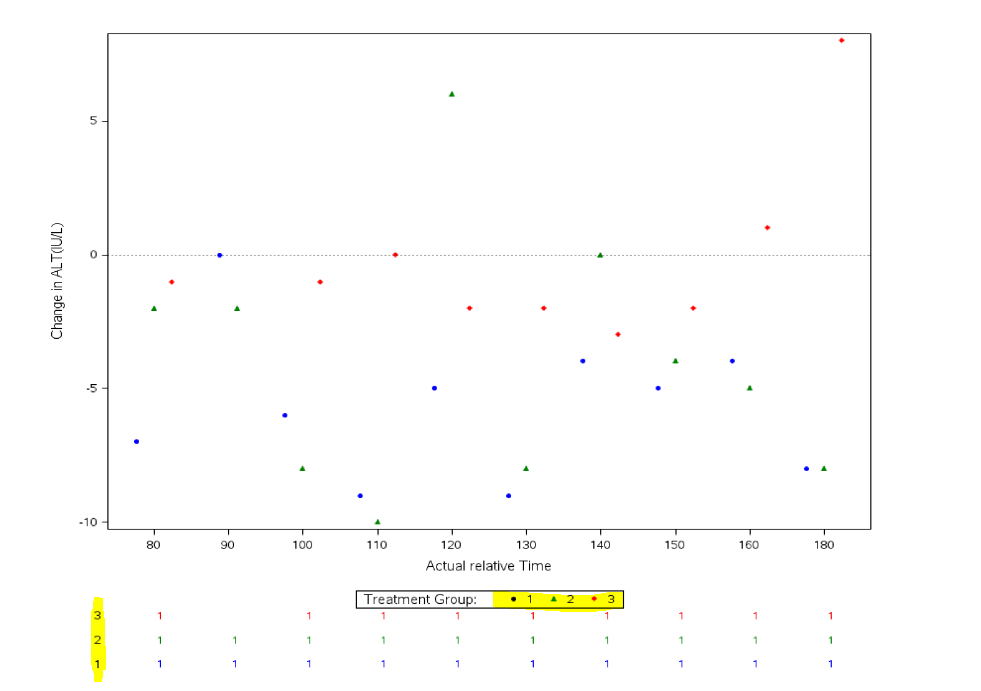## grouporder in proc template does not work

Dear Experts,

This is with regard to the query on sas graph which i am running on sas 9.4. Here i coded the proc template to create a graph.

the graph comes perfectly fine. However the order of the group data in counts table below graph is not in a order, i want it as 1 2 3 and it is in 3 2 1. I tried to use the grouporder option with data, descending and ascending and nothing changes.

could you please suggest any other option by which i will get the order as 1 2 3.

``````proc template;
define statgraph scatterplot;
begingraph;
entrytitle ' ';

discreteattrmap name="lbl" / ignorecase=true;
value  "1" /
markerattrs=GraphData1(color=blue  symbol=circlefilled size=5)
;
value  "2" /
markerattrs=GraphData2(color=green symbol=trianglefilled size=5)
;
value  "3" /
markerattrs=GraphData3(color=red symbol=diamondfilled size=5)
;
enddiscreteattrmap;

discreteattrvar attrvar=lblmarker var=code attrmap="lbl";

layout lattice / columns=1 rows=2 rowweights=(.90 .10);

layout overlay / xaxisopts=( Label="Actual relative Time" type=discrete )
yaxisopts=( Label='Change in ALT(IU/L)' type=linear);
ScatterPlot X=xvar Y=yvar  /  Group=lblmarker GROUPDISPLAY=CLUSTER clusterwidth=0.7 LegendLabel="mean" NAME="SCATTER" ;
referenceline y= 0 / lineattrs=(thickness=1 px color=black pattern=dot);
DiscreteLegend "SCATTER" / border=yes  Location=outside valign=bottom title="Treatment Group:";
endlayout;

layout overlay / walldisplay=none border=false xaxisopts=(display=none type=discrete)
yaxisopts=(Label=' ' type=linear linearopts=(tickvaluesequence=(start=1 end=3 increment=1) viewmin=1 viewmax=3 tickvaluelist=(1 2 3)
tickvalueformat=(extractscale=true)) display=(label tickvalues)) ;
ScatterPlot X=xvarlbl Y=code / Group=lblmarker MARKERCHARACTER=count GROUPORDER=data DATALABELPOSITION=center;
endlayout;
endlayout;
endgraph;
end;
run;

proc sgrender data=test2 template=scatterplot;
run;``````Thanks,
Jag
1 ACCEPTED SOLUTION

Accepted SolutionsJay54
Meteorite | Level 14

## Re: grouporder in proc template does not work

Two points to make here.  With SAS 9.4, it is easier to use AXISTABLE to display the values at the bottom.  See article.  The stacking is done using CLASS variable, and you can also use CLASSORDER to change the order.  For this simple case, SGPLOT is good enough.

In your code, the table is produced by a SCATTER where y=code.  This is not group order, but y order.  You can reverse the y axis for the 2nd cell.  But I would suggest using an AXISTABLE.

4 REPLIES 4Jay54
Meteorite | Level 14

## Re: grouporder in proc template does not work

Two points to make here.  With SAS 9.4, it is easier to use AXISTABLE to display the values at the bottom.  See article.  The stacking is done using CLASS variable, and you can also use CLASSORDER to change the order.  For this simple case, SGPLOT is good enough.

In your code, the table is produced by a SCATTER where y=code.  This is not group order, but y order.  You can reverse the y axis for the 2nd cell.  But I would suggest using an AXISTABLE.

## Re: grouporder in proc template does not work

Thank you Sanjay i agree with your suggestion of using the AXISTABLE. Alsoi followed your second appraoch for the moment by reversing the order of code and it re-ordered the counts data as expected.

In continuation to this thread i would like to check with you if its also possible to put a label as visit count as displayed in the attached image.Thanks,
JagJay54
Meteorite | Level 14

## Re: grouporder in proc template does not work

Yes.  The AXISTABLE has a TITLE option for such purpose.  However, note this axistable title will go under the legend, and will not be drawn vertically like you have shown in the example.

If using a separate Layout Overlay like in your GTL code, the y axis title could be used for this by positoning it at the top of the axis.  See LABELPOSITION inYAXISOPTS in GTL.

## Re: grouporder in proc template does not work

Thank you Sanjay that resolved the issue.
Thanks,
Jag
Discussion stats
• 4 replies
• 1292 views
• 0 likes
• 2 in conversation